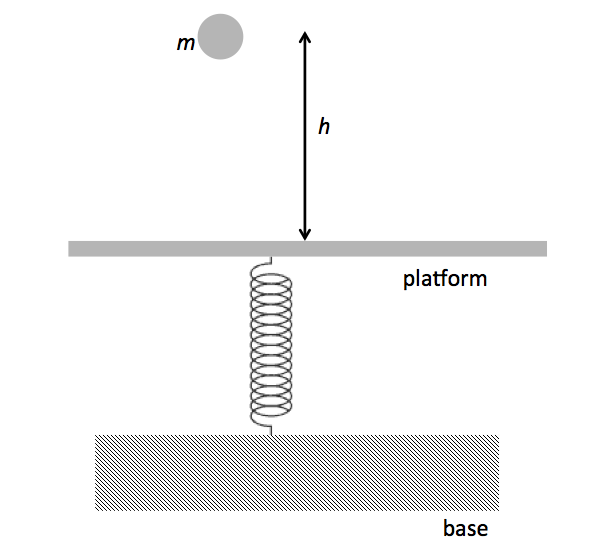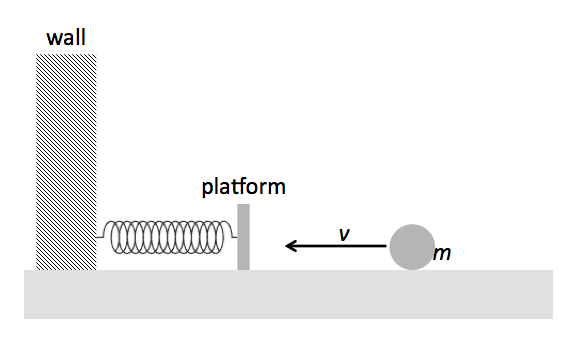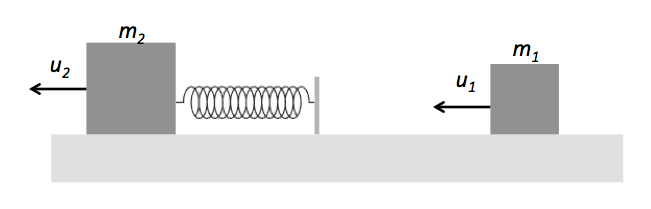Classical Mechanics

# Work 1D - Problem SolvingA body of mass $m = 2 \text{ kg}$ is dropped from $h = 70 \text{ cm}$ above a horizontal platform that is fixed to one end of an elastic spring, as shown in the figure above. As a result the spring is compressed by an amount of $\Delta x = 20 \text{ cm}.$ What is the spring constant of the spring?

The gravitational acceleration is $g= 10 \text{ m/s}^2$ and the air resistance is negligible.

A body initially at rest is dropped from a height of $h = 45\text{ m}.$ If the coefficient of restitution is $e= 0.6,$ what is the height the body reaches at the second bounce?

The gravitational acceleration is $g= 10 \text{ m/s}^2.$

A $14$-meter-long rod of mass $2 \text{ kg}$ with homogeneous density is standing vertically with one end on a horizontal floor. Find the gravitational potential energy of the rod.

The gravitational acceleration is $g = 10 \text{ m/s}^2.$A body of mass $m = 5 \text{ kg}$ is moving on a frictionless horizontal plane with a velocity of $v= 4 \text{ m/s}.$ The body is heading to the vertical platform fixed to one end of an elastic spring, as shown in figure above. As a result the spring is compressed by an amount of $\Delta x = 20 \text{ cm}.$ What is the spring constant of the spring?A block of mass $m_1 = 3 \text{ kg}$ is moving on a frictionless horizontal surface with a velocity of $u_1 = 16 \text{ m/s}$ towards another block of mass $m_2 = 9 \text{ kg}$ that is moving on the same surface with a velocity of $u_2 = 8 \text{ m/s}$ in the same direction. A massless spring of spring constant $k = 36 \text{ N/m}$ is attached to $m_2$ as shown in figure above. When block $m_1$ collides with the spring, what is the maximum change in the spring's length?

×